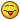## Recommended Posts

Quick question, it's early in the morning here again and my brain has slowed down:

With the torus on the shadows examples in the playground, how would I start its position from another origin but still circle the same point as in the example? If I change the Vector3 numbers it only affects the radius and if I add addition or subtraction to the co-ord it changes its origin but doesn't circle the middle (0,0,0).

##### Share on other sites

Hello, I do not get what you want to achieve##### Share on other sites

http://www.babylonjs-playground.com/#W9S8

Not sure if this is what you want, but take a look this and exam this part of the code:

`// Animations	// Change initial X, Z Position in radians	var alpha = Math.PI;	scene.registerBeforeRender(function () {		torus.rotation.x += 0.01;		torus.rotation.z += 0.02;		// radius of circle path		var radius = 30;		// Center Rotation Axis (x0, 10, z0)		var x0 = 10;		var z0 = 10; 				torus.position = new BABYLON.Vector3(x0 + Math.cos(alpha) * radius, 10, z0 + Math.sin(alpha) * radius);		alpha += 0.01;	});`

I added variables to be able to change initial conditions. If this does not help, please explain further.

Thanks!

##### Share on other sites

sorry for not explaining too clearly. Amorgan the code you have shown is the same code I am trying to adjust, as mentioned (but not very clearly) If I add those variables (x0 and z0) it changes the starting position of the torus however it doesn't circle the same spot.

I've made a diagram to explain things as it will be a lot clearer##### Share on other sites

So if you take a look back, you can see I added a comment:

`// Change initial X, Z Position in radiansvar alpha = Math.PI;`

This will adjust your starting position. I am not sure how familiar you are with radians, but since this example is set to convert polar coordinates to cartesian, alpha represents the angle at which the object will start.

In your diagram, alpha = 0, is where it is set to load. Where you want it to load would be when alpha is 3*Math.PI/2.

In short:

alpha = 0 radians = 0 degrees

alpha = Math.PI/2 radian = 90 degrees

alpha = Math.PI radians = 180 degrees

alpha = 3*Math.PI/2 radians = 270 degrees (the one you want in this example)

alpha = 2*Math.PI radians = 360 degrees = 0 degrees

Hope this helps

##### Share on other sites

It adjusts the starting position but it also adjusts the center of which it rotates around (so no longer circling around the very center of the ground).

*edit (oh I see the initialisation of the alpha will do this) thanks! (I still had x0 = 10 and z0 = 10 which is why it was no longer rotating around the centre)

## Join the conversation

You can post now and register later. If you have an account, sign in now to post with your account.
Note: Your post will require moderator approval before it will be visible.×   Pasted as rich text.   Paste as plain text instead

Only 75 emoji are allowed.